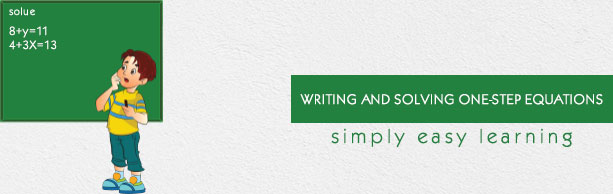# Writing and Solving One-Step Equations

This tutorial provides comprehensive coverage of writing and solving one-step equations based on Common Core (CCSS) and State Standards and its prerequisites. Students can navigate learning paths based on their level of readiness. Institutional users may customize the scope and sequence to meet curricular needs. This simple tutorial uses appropriate examples to help you understand writing and solving one-step equations in a general and quick way.

# Audience

This tutorial has been prepared for beginners to help them understand the basics of writing and solving one-step equations. After completing this tutorial, you will find yourself at a moderate level of expertise in writing and solving one-step equations, from where you can advance further.

# Prerequisites

Before proceeding with this tutorial, you need a basic knowledge of elementary math concepts such number sense, addition, subtraction, multiplication, division, multiples and factors of numbers, variables, constants, terms, expressions, equations and so on.